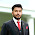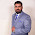## Simple Tic Tac Toe

Given below is a code for simple tic tac toe game made using do while loops....
Its really very simple... Just pay close attention to the code and comments .. If you still do not understand you can ask in comments about it :)

Output:

Code:

```// www.cppfuzz.com (Ahmad Mukhtar)
/*
______ .______   .______    _______  __    __   ________   ________
/      ||   _  \  |   _  \  |   ____||  |  |  | |       /  |       /
|  ,----'|  |_)  | |  |_)  | |  |__   |  |  |  | `---/  /   `---/  /
|  |     |   ___/  |   ___/  |   __|  |  |  |  |    /  /       /  /
|  `----.|  |      |  |      |  |     |  `--'  |   /  /----.  /  /----.
\______|| _|      | _|      |__|      \______/   /________| /________|

*/
#include <iostream>
#include <conio.h>
using namespace std;

void main() {
char square1='1';
char square2='2';
char square3='3';
char square4='4';
char square5='5';
char square6='6';
char square7='7';
char square8='8';
char square9='9';
int playerturn=1;
bool gameover=true;

// Loop for game starts here...
do {
// This loop prints the board.
cout << square1 << "|" << square2 << "|" << square3 << endl;
cout << "-+-+-"<< endl;
cout << square4 << "|" << square5 << "|" << square6 << endl;
cout << "-+-+-"<< endl;
cout << square7 << "|" << square8 << "|" << square9 << endl;

// It sets X for Player 1 and O for Player 2
char playermark;
if (playerturn == 1) {
playermark = 'X';
} else {
playermark = 'O';
}

// It asks the user for move
cout << "Player" << playerturn << "'s move:" << endl;
bool validmove;
// This loop continues until a valid move is given
do {
char nextmove;
cin >> nextmove;
validmove = true;
system("cls");
// Check for a valid move
if (nextmove == '1' && square1 == '1') {
square1 = playermark;
} else if (nextmove == '2' && square2 == '2') {
square2 = playermark;
} else if (nextmove == '3' && square3 == '3') {
square3 = playermark;
} else if (nextmove == '4' && square4 == '4') {
square4 = playermark;
} else if (nextmove == '5' && square5 == '5') {
square5 = playermark;
} else if (nextmove == '6' && square6 == '6') {
square6 = playermark;
} else if (nextmove == '7' && square7 == '7') {
square7 = playermark;
} else if (nextmove == '8' && square8 == '8') {
square8 = playermark;
} else if (nextmove == '9' && square9 == '9') {
square9 = playermark;
} else {
cout << "Invalid Move. Try again." << endl;
validmove = false;
}
} while (!validmove);

gameover  = false;
bool wingame = true;
// It checks the winning conditions
if (square1 != '1') {
if (square2 == square1 && square3 == square1) {
gameover = true;
}
if (square4 == square1 && square7 == square1) {
gameover = true;
}
}
if (square5 != '5') {
if (square1 == square5 && square9 == square5) {
gameover = true;
}
if (square2 == square5 && square8 == square5) {
gameover = true;
}
if (square4 == square5 && square6 == square5) {
gameover = true;
}
if (square3 == square5 && square7 == square5) {
gameover = true;
}
}
if (square9 != '9') {
if (square3 == square9 && square6 == square9) {
gameover = true;
}
if (square7 == square9 && square8 == square9) {
gameover = true;
}
}
// It will check for draw conditions
if (square1 != '1' && square2 != '2' && square3 != '3' &&
square4 != '4' && square5 != '5' && square6 != '6' &&
square7 != '7' && square8 != '8' && square9 != '9' && !gameover)
{
gameover = true;
wingame = false;
}
if (gameover) {
if (wingame==true) {
cout << "Player" << playerturn << " wins..." << endl;
}
if (wingame==false) {
cout << "Draw Game." << endl;
}
// Final shape of board after getting all the moves till end of game
cout << square1 << "|" << square2 << "|" << square3 << endl;
cout << "-+-+-"<< endl;
cout << square4 << "|" << square5 << "|" << square6 << endl;
cout << "-+-+-"<< endl;
cout << square7 << "|" << square8 << "|" << square9 << endl;

cout << "Game Over" << endl;
cout << "Do you want to play again (y/n)?" << endl;
char playagain;
cin >> playagain;

if (playagain == 'y') {
system("cls");
gameover = false;
// resets the board
square1 = '1';
square2 = '2';
square3 = '3';
square4 = '4';
square5 = '5';
square6 = '6';
square7 = '7';
square8 = '8';
square9 = '9';
}
playerturn = 1;
} else {
// Alternate player turns
if (playerturn == 1) {
playerturn = 2;
} else {
playerturn = 1;
}
}
} while (!gameover);
}```

1.1.